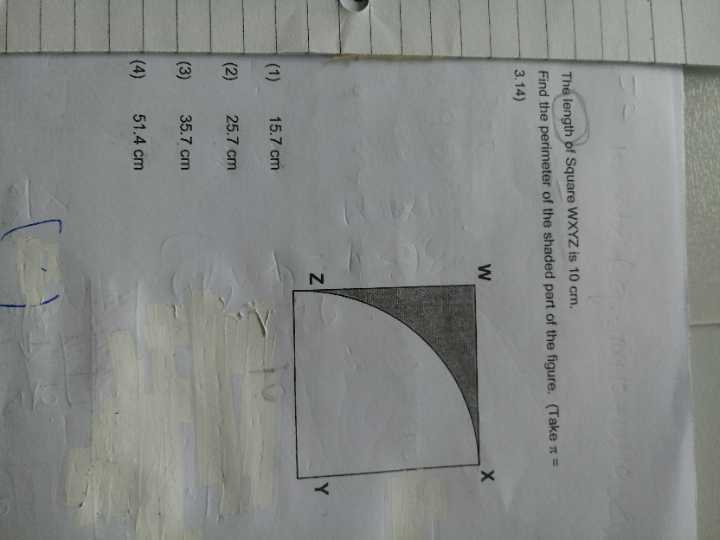# QuestionPls help.
Try many times…cannot find the answer

Length of square = radius of circle

Circumference of circle = 2 π r = (2 x 3.14 x 10) cm = 62.8 cm

Length of quarter arc of the circle = 62.8/4 = 15.7 cm

Total perimeter of shaded area = 15.7 cm + 10cm + 10 cm = 35.7 cm (Ans)

0 Replies 0 Likes